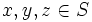# Orbital maximin equals size of set for abelian groups

Jump to: navigation, search

## Statement

Suppose$n$ is a natural number greater than$1$. Then, for any abelian group$G$ acting on a set of size$n$, the maximum possible value of the minimum of the sizes of the orbitals is$n$. Further, there exists an abelian group (namely, the cyclic group of order$n$) for which this maximum is achieved.

This is a resolution to the Orbital maximin problem (?) for abelian groups.

## Proof

We assume that$n$ is at least$3$. The result holds trivially for$n = 2$.

### Reducing to the case of a transitive action

Suppose the action of$G$ on a set$S$ of size$n$ has more than one orbit. Let$m$ be the size of the smallest orbit, which we call$\mathcal{O}$.$G$ restricts to a permutation action on$\mathcal{O}$. In particular, the orbit of any orbital with both points in$\mathcal{O}$ lies completely inside the orbital set for$\mathcal{O}$.

Thus, if we prove the result for all$m$ for a transitive action, then using$m \le n$ would complete the proof. We can thus assume that the action is transitive.

### Every element must act freely (semiregularly), i.e., without fixed points

We assume$G$ acts transitively on a set$S$ of size$n$.

Suppose we have an element$\sigma$ in$G$ and elements$x,y,z \in S$ such that$\sigma(x) = x$ and$\sigma(y) = z$. Since$G$ acts transitively, there exists$\tau$ such that$\tau(x) = y$. Then,$\tau(\sigma(x)) = \tau(x) = y$ whereas$\sigma(\tau(x)) = \sigma(y) = z$. Thus,$\sigma \circ \tau \ne \tau \circ \sigma$, contradicting abelianness.

Note that we can in fact deduce that the action must be a regular group action since it is both transitive and semiregular. This is part of a more general idea: any action that centralizes a transitive group action must be semiregular.

### The size of an orbital is exactly$n$ for a transitive action

As before,$G$ acts transitively on a set$S$ of size$n$.

Consider any pair$(x,y), x \ne y$. We know that for any two elements$\sigma_1, \sigma_2$, if$\sigma_1(x) = \sigma_2(x)$, then$\sigma_2^{-1}(\sigma_1(x)) = x$. Since the action is free, this forces$\sigma_1 = \sigma_2$, also forcing$\sigma_1(y) = \sigma_2(y)$. Thus, no two distinct elements of the orbital of$(x,y)$ can have the same first coordinate. Since the number of first coordinates is$n$, we obtain that the size of the orbital is exactly$n$.